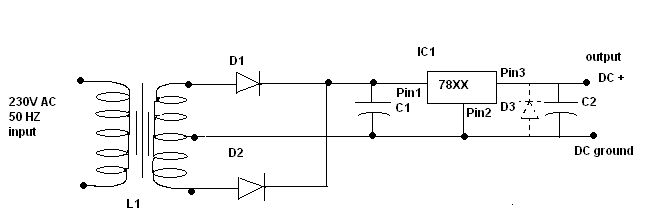Design Guide Details
Design Guide Details
Date: 03-01-17

# DC power supply circuits

From simple LED light to embedded boards all need clean regulated DC power supply. Here we give few schematic diagrams to build power supply circuits from simple to complex types. If power consumption is a critical factor go for switching only.

Here is one most popular, old, reliable and simple circuit for fixed DC power supply. These are ideal for circuits with an average current requirement of less than 1.00 amp.

5V/9V/12V fixed power supply (Positive) at 1 Ampere current ratingCircuit Diagram (Schematic Diagram)-1

L1 = Step down transformer with i/p of 230 AC 50 Hz and output of (XX ) - 0- (XX)) volts(rms).
XX  = Required DC output voltage.

Here is the table for different voltages

 Output voltage (DC Volts) Transformer rating (rms Volts) 5 230: 5-0-5 9 230:9-0-9 12 230:12-0-12 15 230:15-0-15

The current rating has to be more than 1 Amp.

D1, D2 = Diodes 1N4003
D3 = Diode 1N4003/ 1N4001 (optional)
C1 = 1000 Micro Farad aluminum electrolytic capacitor (For loads less than 100mA you can sustitute with 220 microfards capacitor), Voltage rating = 2.5 times of Output Voltage.
C2 = 10 Micro Farad aluminum electrolytic capacitor
IC1 = 7805 for + 5V DC output
=7809 for +9V DC output
=7812 for +12V DC output
=7815 for +15V DC output

5V/9V/12V fixed power supply (Negative) at 1 Ampere current ratingCircuit Diagram (Schematic Diagram)-2

L1 = Step down transformer with i/p of 230 AC 50 Hz and output of (XX ) - 0- (XX)) volts(rms).
XX  = Required DC output voltages.

 Output voltage (DC Volts) Transformer rating (rms Volts) 5 230: 5-0-5 9 230:9-0-9 12 230:12-0-12 15 230:15-0-15

The current rating has to be more than 1 Amp.

D1, D2 = Diodes 1N4003
D3 = Diode 1N4003/ 1N4001 (optional)
C1 = 1000 Micro Farad aluminum electrolytic capacitor(For loads less than 100mA you can sustitute with 220 microfards capacitor). Voltage rating = 2.5 times of Output Voltage.
C2 = 10 Micro Farad aluminum electrolytic capacitor
IC1 = 7905 for -5V DC output
=7909 for -9V DC output
=7912 for -12V DC output
=7915 for -15V DC output